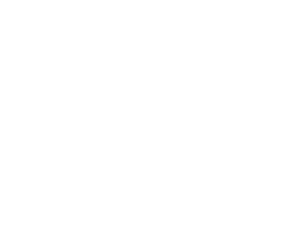Please click on one of the buttons below to use one of our calculators.

##Weight CalculatorConversion Calculators

Industrial Metal Supply provides an online metal weight calculator to help customers quickly and easily figure out how much any amount of metal will weigh. It will work for more than 20 types of metal and alloys and can calculate any quantity you need. Customers use it to figure out shipping costs, determine whether a truck or trailer can handle the amount of weight that is being moved, and for weight bearing projects.

## Metal Weight Calculation Formula

The basic metal weight calculation formula is the same for any type, size or shape of metal. First, you calculate the volume. For a rectangular piece of metal, the formula for volume is:

V = Width x Height x Length

Other shapes, such as tubes or circles, have more complicated formulas for volume. Once you know the volume of your metal you multiply it by the density, which is the weight of the material per unit volume. Each type or alloy of metal has a unique density. For example, the density of Stainless 300 Series is 0.0078 g/mm3.

You can find the density of your metal or alloy by searching online or looking at a metal reference chart. The IMS Metal Calculator includes density data for each type of metal we sell, so you don’t need to look it up.

For any type of mathematical calculation, it’s important to keep the units of measurement the same, whether Metric or English. The IMS Metal Calculator keeps it simple by allowing you to choose different units for each dimension you enter, based on your order. It then automatically converts them to the correct units.

## Calculating The Weight of Metal

Finally, to calculate the weight of your metal, multiply the volume times the density times any conversion factors:

Weight = Volume x Density x Conversion Factor

## Our Metal Weight Calculator Makes it Easy

It can be tricky to calculate metal weight, but our online calculator makes it simple. Follow these basic steps to take the guesswork out of the process:
1. Select the metal type you are working with from the drop down menu, for example, Aluminum 6063 or Brass.
2. Choose the shape of the piece from the drop down menu.
3. Type in the number of pieces you are purchasing. Note: You must calculate each different shape and size separately, and then add the results together by hand.
4. Next, enter the dimensions of the piece. This will change depending on the shape you select. For example, if you choose a circular shape, you will be asked to enter the diameter and length. For a tubular piece, you will need to enter the outer diameter, wall thickness, and length.
5. Select the units for each dimension from the drop down menu.
6. Hit the Calculate button. All results are reported in pounds.
Note: IMS also provides an online conversion calculator here.